# RD Sharma Solutions for Class 12 Maths Exercise 15.1 Chapter 15 Mean Value Theorems

RD Sharma Solutions for Class 12 Maths Exercise 15.1 Chapter 15 Mean Value Theorems are provided here. Rolle’s theorem and the geometrical theorem of Rolle’s theorem of given functions with illustrative examples are the main topics which are explained in Exercise 15.1. The exercise-wise solutions are prepared by our experts with explanations for each step to make it easier for students while solving problems. It helps to check their knowledge of the concepts, which are discussed in this chapter.

In order to master Maths, students can use RD Sharma Solutions for Class 12 Maths Chapter 15 Mean Value Theorems Exercise 15.1 as their reference material. Some of the essential topics of this exercise are enlisted here.

• Rolle’s theorem
• Geometrical interpretation of Rolle’s theorem
• Algebraic interpretation of Rolle’s theorem
• Checking the applicability of Rolle’s theorem
• Verification of Rolle’s theorem for a given function defined on a given interval
• Miscellaneous applications of Rolle’s theorem

## RD Sharma Solutions for Class 12 Maths Chapter 15 Mean Value Theorems Exercise 15.1

### Access Another Exercise of RD Sharma Solutions for Class 12 Maths Chapter 15 – Mean Value Theorems

Exercise 15.1 Page No: 15.9

1. Discuss the applicability of Rolle’s Theorem for the following functions on the indicated intervals: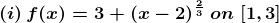Solution: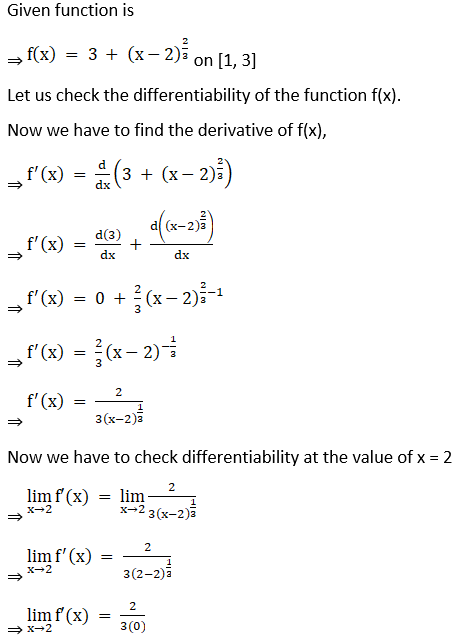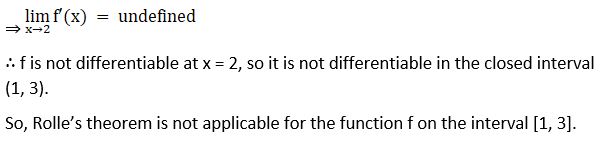(ii) f (x) = [x] for -1 ≤ x ≤ 1, where [x] denotes the greatest integer not exceeding x

Solution: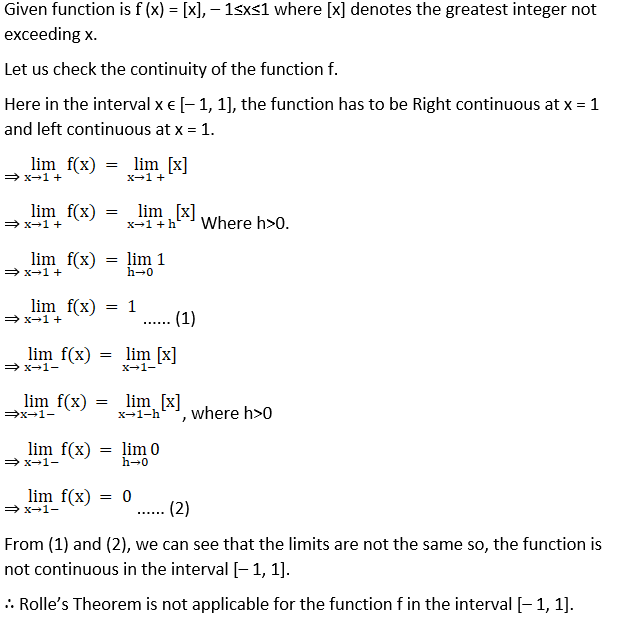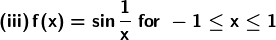Solution: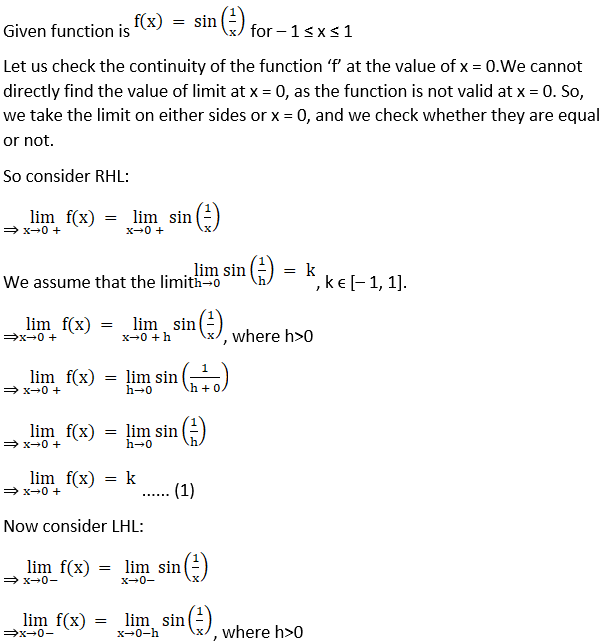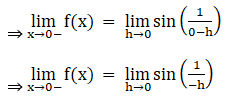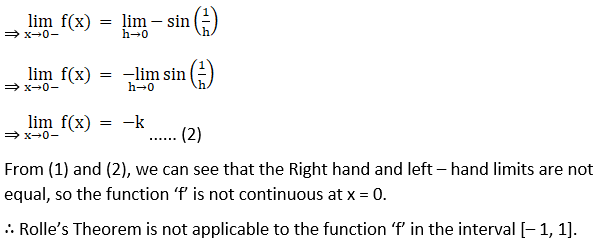(iv) f (x) = 2x2 – 5x + 3 on [1, 3]

Solution:

Given function is f (x) = 2x2 – 5x + 3 on [1, 3]

Since the given function f is a polynomial, it is continuous and differentiable everywhere.

Now, we find the values of the function at the extreme values.

⇒ f (1) = 2(1)2–5(1) + 3

⇒ f (1) = 2 – 5 + 3

⇒ f (1) = 0…… (1)

⇒ f (3) = 2(3)2–5(3) + 3

⇒ f (3) = 2(9)–15 + 3

⇒ f (3) = 18 – 12

⇒ f (3) = 6…… (2)

From (1) and (2), we can say that f (1) ≠ f (3)

∴ Rolle’s Theorem is not applicable for the function f in interval [1, 3].

(v) f (x) = x2/3 on [-1, 1]

Solution: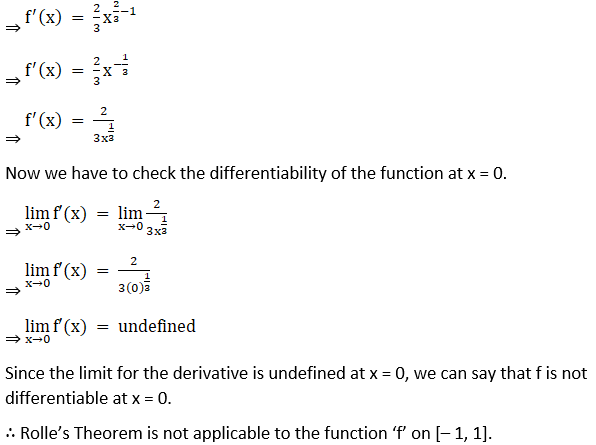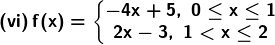Solution: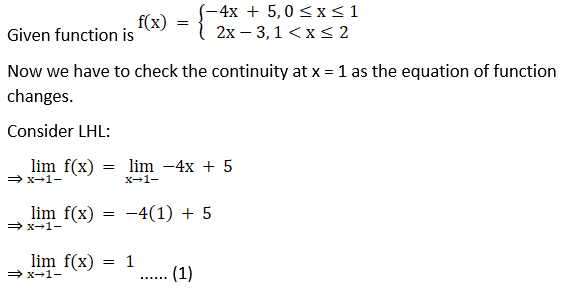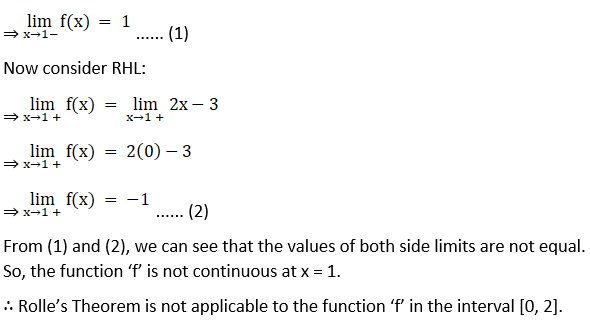2. Verify the Rolle’s Theorem for each of the following functions on the indicated intervals:

(i) f (x) = x2 – 8x + 12 on [2, 6]

Solution:

Given function is f (x) = x2 – 8x + 12 on [2, 6]

Since the given function f is a polynomial, it is continuous and differentiable everywhere, i.e., on R.

Let us find the values at extremes:

⇒ f (2) = 22 – 8(2) + 12

⇒ f (2) = 4 – 16 + 12

⇒ f (2) = 0

⇒ f (6) = 62 – 8(6) + 12

⇒ f (6) = 36 – 48 + 12

⇒ f (6) = 0

∴ f (2) = f(6), Rolle’s theorem is applicable for function f on [2,6].

Now we have to find the derivative of f(x)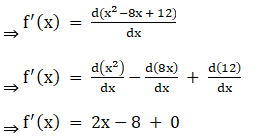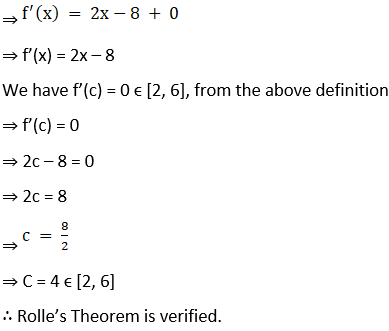(ii) f(x) = x2 – 4x + 3 on [1, 3]

Solution:

Given function is f (x) = x2 – 4x + 3 on [1, 3]

Since, given function f is a polynomial, it is continuous and differentiable everywhere, i.e., on R. Let us find the values at extremes:

⇒ f (1) = 12 – 4(1) + 3

⇒ f (1) = 1 – 4 + 3

⇒ f (1) = 0

⇒ f (3) = 32 – 4(3) + 3

⇒ f (3) = 9 – 12 + 3

⇒ f (3) = 0

∴ f (1) = f(3), Rolle’s theorem is applicable for function ‘f’ on [1,3].

Let’s find the derivative of f(x)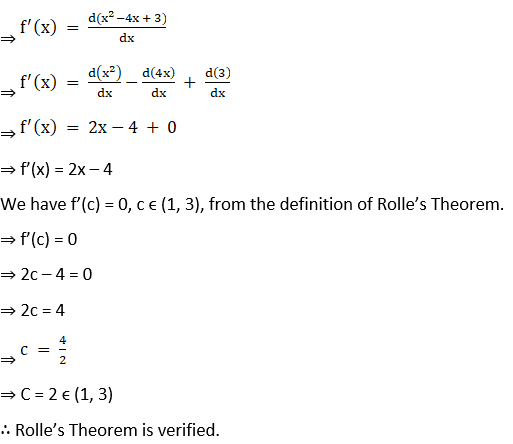⇒ f’(x) = 2x – 4

We have f’(c) = 0, c ϵ (1, 3), from the definition of Rolle’s Theorem.

⇒ f’(c) = 0

⇒ 2c – 4 = 0

⇒ 2c = 4

⇒ c = 4/2

⇒ C = 2 ϵ (1, 3)

∴ Rolle’s Theorem is verified.

(iii) f (x) = (x – 1) (x – 2)2 on [1, 2]

Solution:

Given function is f (x) = (x – 1) (x – 2)2 on [1, 2]

Since the given function f is a polynomial, it is continuous and differentiable everywhere that is on R.

Let us find the values at extremes:

⇒ f (1) = (1 – 1) (1 – 2)2

⇒ f (1) = 0(1)2

⇒ f (1) = 0

⇒ f (2) = (2 – 1)(2 – 2)2

⇒ f (2) = 02

⇒ f (2) = 0

∴ f (1) = f (2), Rolle’s Theorem applicable for function ‘f’ on [1, 2].

Let’s find the derivative of f(x)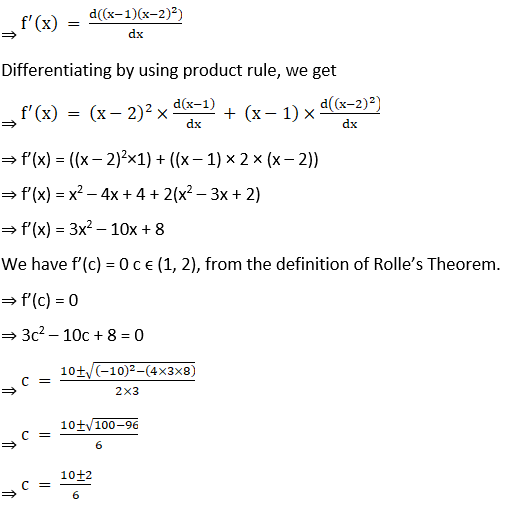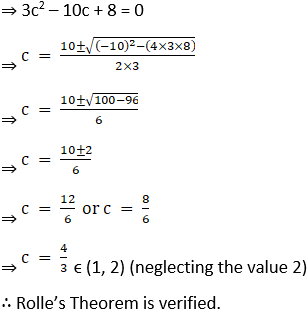(iv) f (x) = x (x – 1)2 on [0, 1]

Solution:

Given function is f (x) = x(x – 1)2 on [0, 1]

Since the given function f is a polynomial, it is continuous and differentiable everywhere, that is, on R.

Let us find the values at extremes

⇒ f (0) = 0 (0 – 1)2

⇒ f (0) = 0

⇒ f (1) = 1 (1 – 1)2

⇒ f (1) = 02

⇒ f (1) = 0

∴ f (0) = f (1), Rolle’s theorem applicable for function ‘f’ on [0,1].

Let’s find the derivative of f(x)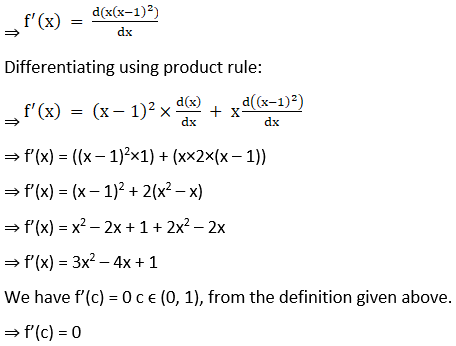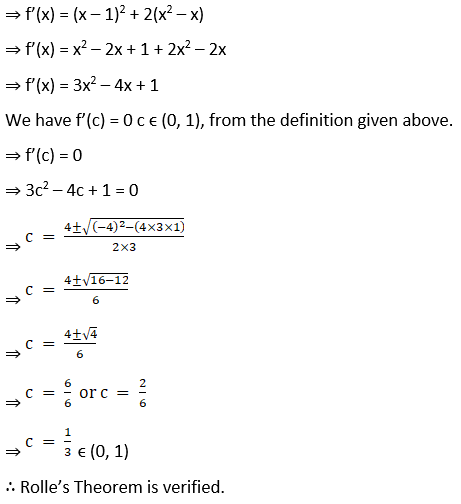(v) f (x) = (x2 – 1) (x – 2) on [-1, 2]

Solution:

Given function is f (x) = (x2 – 1) (x – 2) on [– 1, 2]

Since the given function f is a polynomial, it is continuous and differentiable everywhere, that is, on R.

Let us find the values at extremes:

⇒ f ( – 1) = (( – 1)2 – 1)( – 1 – 2)

⇒ f ( – 1) = (1 – 1)( – 3)

⇒ f ( – 1) = (0)( – 3)

⇒ f ( – 1) = 0

⇒ f (2) = (22 – 1)(2 – 2)

⇒ f (2) = (4 – 1)(0)

⇒ f (2) = 0

∴ f (– 1) = f (2), Rolle’s theorem applicable for function f on [ – 1,2].

Let’s find the derivative of f(x)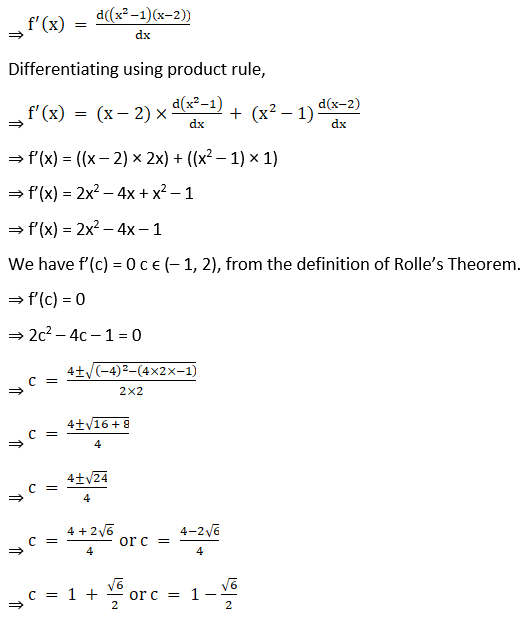f’(x) = 3x2 – 4x – 1

We have f’(c) = 0 c ∈ (-1, 2), from the definition of Rolle’s Theorem

f'(c) = 0

3c2 – 4c – 1 = 0

c = 4 ± √[(-4)2 – (4 x 3 x -1)]/ (2 x 3) [Using the Quadratic Formula]

c = 4 ± √[16 + 12]/ 6

c = (4 ± √28)/ 6

c = (4 ± 2√7)/ 6

c = (2 ±√7)/ 3 = 1.5 ± √7/3

c = 1.5 + √7/3 or 1.5 – √7/3

So,

c = 1.5 – √7/3 since c ∈ (-1, 2)

∴ Rolle’s Theorem is verified.

(vi) f (x) = x (x – 4)2 on [0, 4]

Solution:

Given function is f (x) = x (x – 4)2 on [0, 4]

Since the given function f is a polynomial, it is continuous and differentiable everywhere, i.e., on R.

Let us find the values at extremes:

⇒ f (0) = 0(0 – 4)2

⇒ f (0) = 0

⇒ f (4) = 4(4 – 4)2

⇒ f (4) = 4(0)2

⇒ f (4) = 0

∴ f (0) = f (4), Rolle’s theorem is applicable for function ‘f’ on [0,4].

Let’s find the derivative of f(x):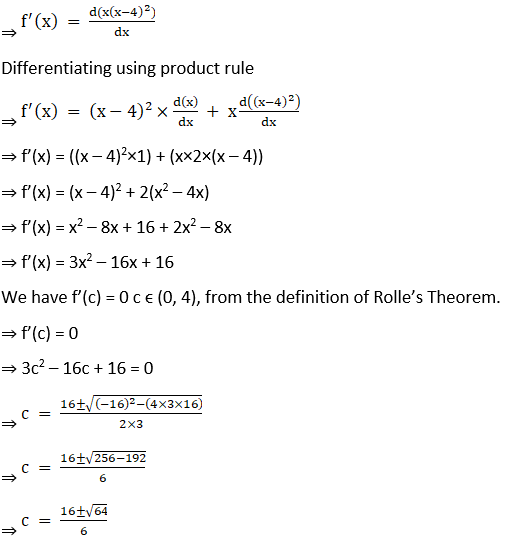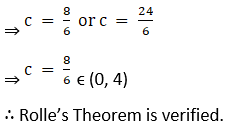(vii) f (x) = x (x – 2)2 on [0, 2]

Solution:

Given function is f (x) = x (x – 2)2 on [0, 2]

Since the given function f is a polynomial, it is continuous and differentiable everywhere that is on R.

Let us find the values at extremes:

⇒ f (0) = 0(0 – 2)2

⇒ f (0) = 0

⇒ f (2) = 2(2 – 2)2

⇒ f (2) = 2(0)2

⇒ f (2) = 0

f (0) = f(2), Rolle’s theorem is applicable for function f on [0,2].

Let’s find the derivative of f(x)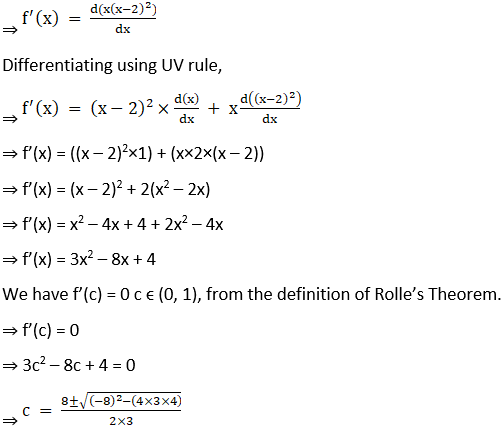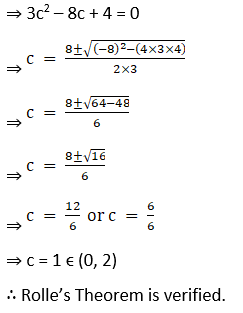c = 12/6 or 4/6

c = 2 or 2/3

So,

c = 2/3 since c ∈ (0, 2)

∴ Rolle’s Theorem is verified.

(viii) f (x) = x2 + 5x + 6 on [-3, -2]

Solution:

Given function is f (x) = x2 + 5x + 6 on [– 3, – 2]

Since the given function f is a polynomial, it is continuous and differentiable everywhere, i.e., on R. Let us find the values at extremes:

⇒ f ( – 3) = ( – 3)2 + 5( – 3) + 6

⇒ f ( – 3) = 9 – 15 + 6

⇒ f ( – 3) = 0

⇒ f ( – 2) = ( – 2)2 + 5( – 2) + 6

⇒ f ( – 2) = 4 – 10 + 6

⇒ f ( – 2) = 0

∴ f (– 3) = f( – 2), Rolle’s theorem applicable for function f on [ – 3, – 2].

Let’s find the derivative of f(x):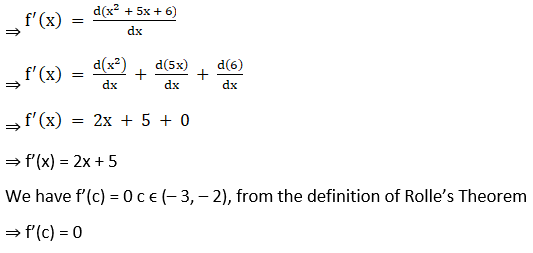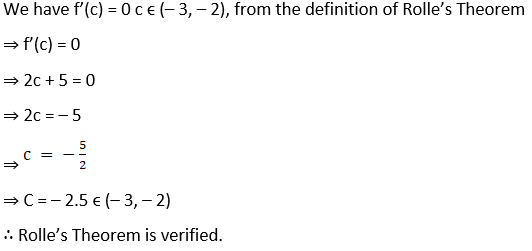3. Verify the Rolle’s Theorem for each of the following functions on the indicated intervals:

(i) f (x) = cos 2 (x – π/4) on [0, π/2]

Solution: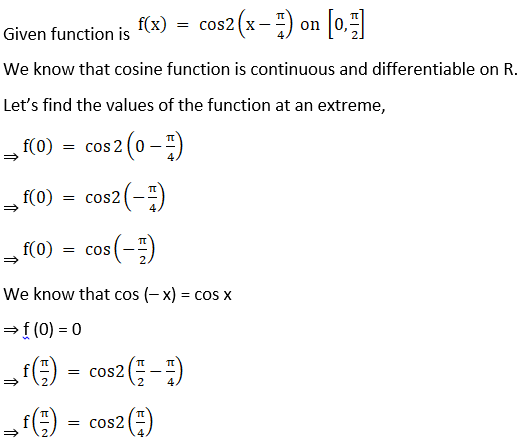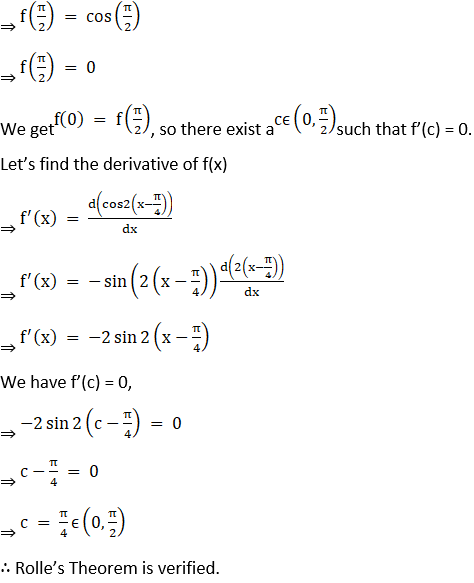(ii) f (x) = sin 2x on [0, π/2]

Solution: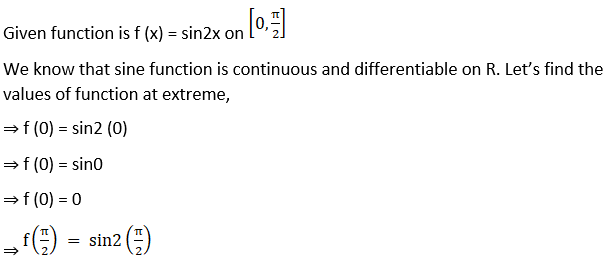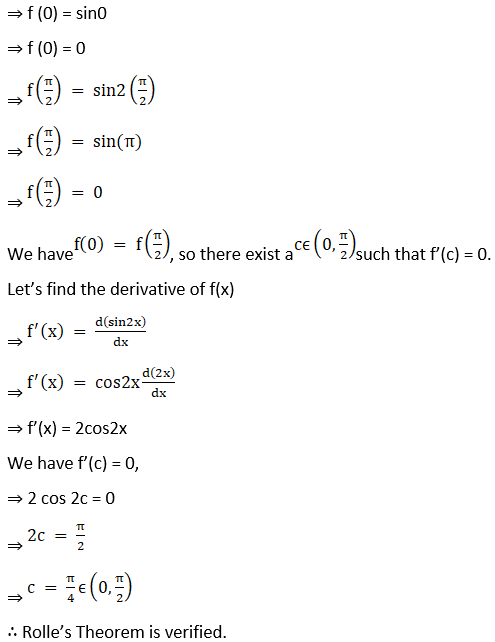(iii) f (x) = cos 2x on [-π/4, π/4]

Solution: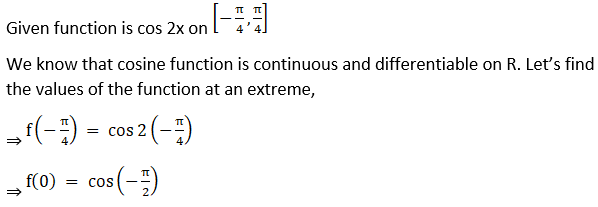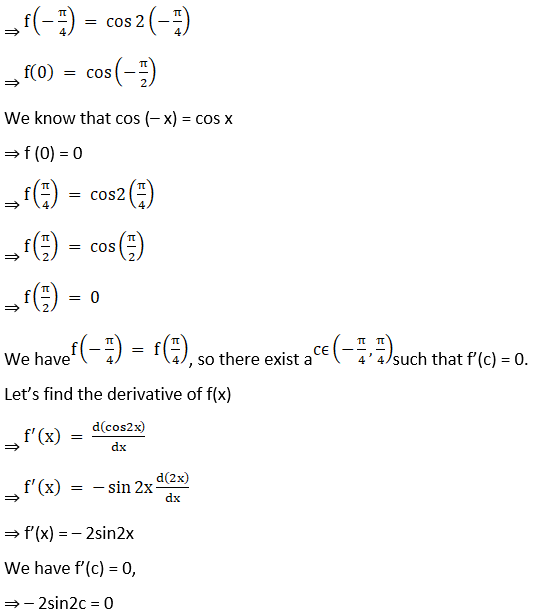sin 2c = 0

⇒ 2c = 0

So,

c = 0 as c ∈ (-π/4, π/4)

∴ Rolle’s Theorem is verified.

(iv) f (x) = ex sin x on [0, π]

Solution:

Given function is f (x) = ex sin x on [0, π]

We know that exponential and sine functions are continuous and differentiable on R. Let’s find the values of the function at an extreme,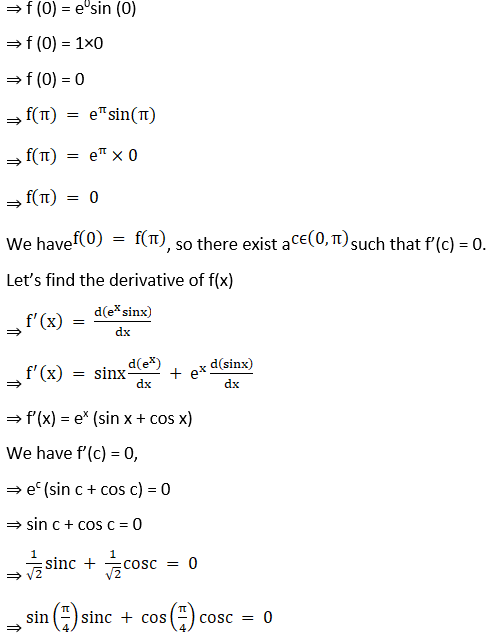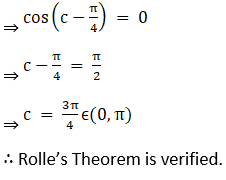(v) f (x) = ex cos x on [-π/2, π/2]

Solution: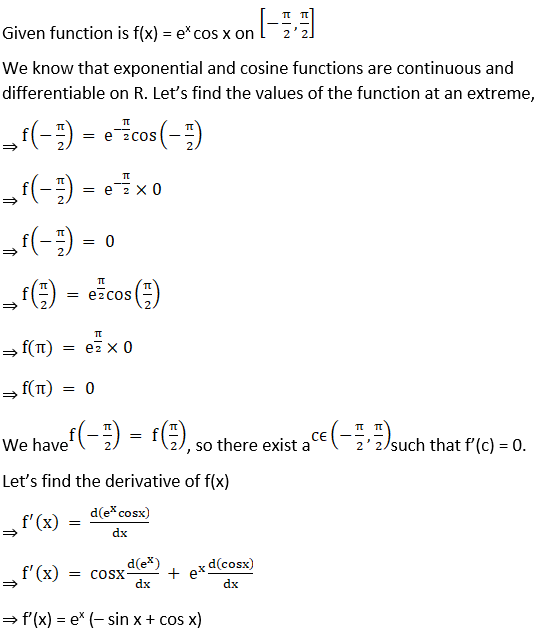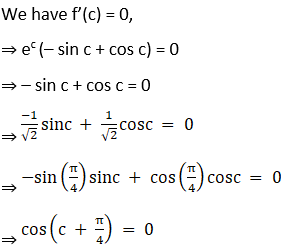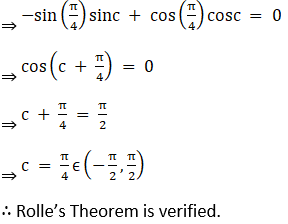(vi) f (x) = cos 2x on [0, π]

Solution:

Given function is f (x) = cos 2x on [0, π]

We know that the cosine function is continuous and differentiable on R. Let’s find the values of the function at extreme,

⇒ f (0) = cos2(0)

⇒ f (0) = cos(0)

⇒ f (0) = 1

⇒ f (π) = cos2(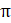)

⇒ f (π) = cos(2 π)

⇒ f (π) = 1

We have f (0) = f (π), so there exists a c belonging to (0, π) such that f’(c) = 0.

Let’s find the derivative of f(x)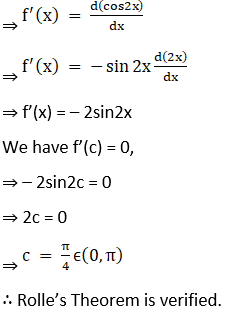sin 2c = 0

So, 2c = 0 or π

c = 0 or π/2

But,

c = π/2 as c ∈ (0, π)

Hence, Rolle’s Theorem is verified.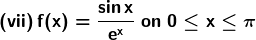Solution: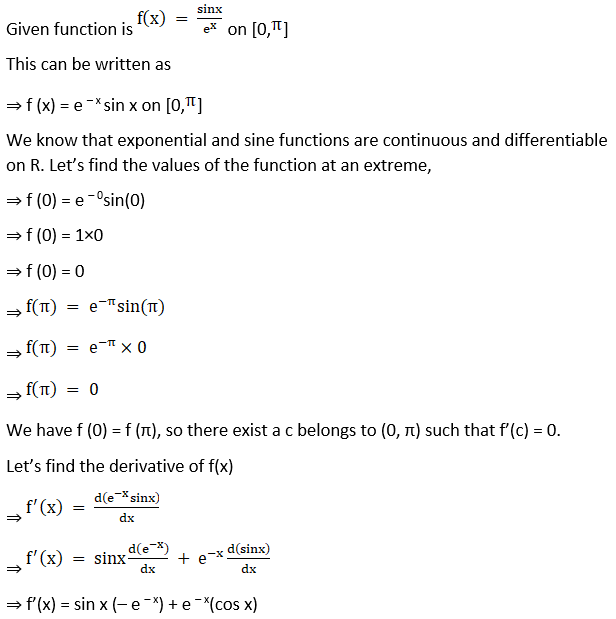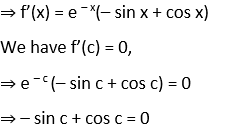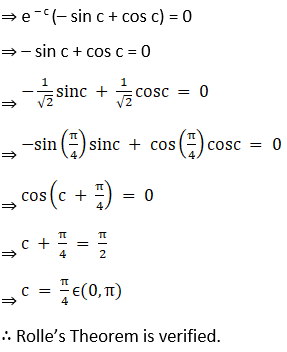(viii) f (x) = sin 3x on [0, π]

Solution:

Given function is f (x) = sin3x on [0, π]

We know that the sine function is continuous and differentiable on R. Let’s find the values of the function at extreme,

⇒ f (0) = sin3(0)

⇒ f (0) = sin0

⇒ f (0) = 0

⇒ f (π) = sin3(π)

⇒ f (π) = sin(3 π)

⇒ f (π) = 0

We have f (0) = f (π), so there exists a c belonging to (0, π) such that f’(c) = 0.

Let’s find the derivative of f(x)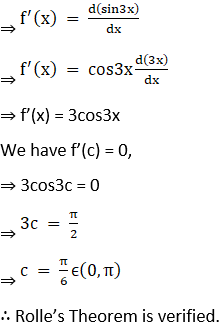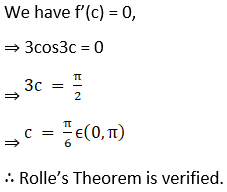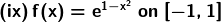Solution: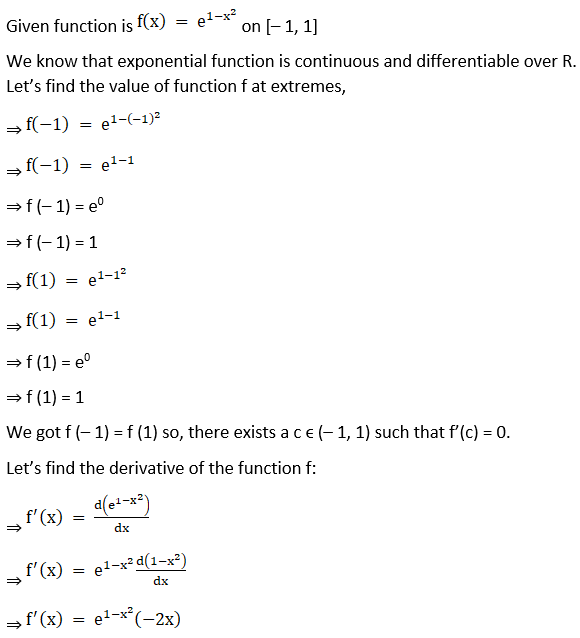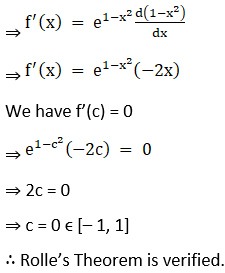(x) f (x) = log (x2 + 2) – log 3 on [-1, 1]

Solution:

Given function is f (x) = log(x2 + 2) – log3 on [– 1, 1]

We know that logarithmic function is continuous and differentiable in its own domain. We check the values of the function at the extreme,

⇒ f (– 1) = log((– 1)2 + 2) – log 3

⇒ f (– 1) = log (1 + 2) – log 3

⇒ f (– 1) = log 3 – log 3

⇒ f ( – 1) = 0

⇒ f (1) = log (12 + 2) – log 3

⇒ f (1) = log (1 + 2) – log 3

⇒ f (1) = log 3 – log 3

⇒ f (1) = 0

We have got f (– 1) = f (1). So, there exists a c such that c ϵ (– 1, 1) such that f’(c) = 0.

Let’s find the derivative of the function f,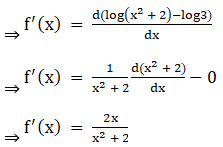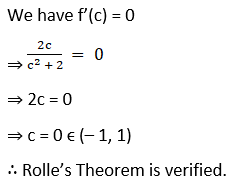(xi) f (x) = sin x + cos x on [0, π/2]

Solution: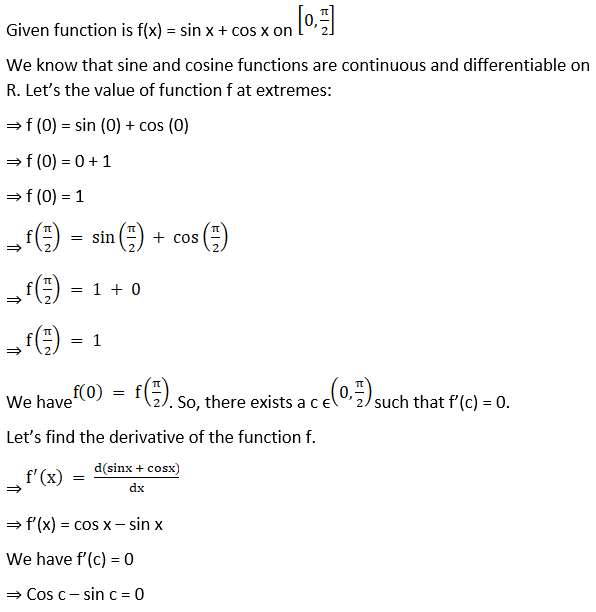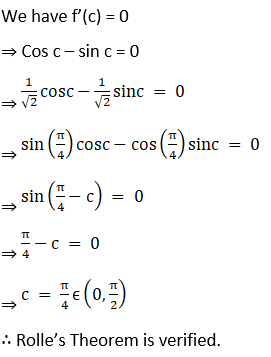(xii) f (x) = 2 sin x + sin 2x on [0, π]

Solution:

Given function is f (x) = 2sinx + sin2x on [0, π]

We know that the sine function is continuous and differentiable over R.

Let’s check the values of function f at the extremes

⇒ f (0) = 2sin(0) + sin2(0)

⇒ f (0) = 2(0) + 0

⇒ f (0) = 0

⇒ f (π) = 2sin(π) + sin2(π)

⇒ f (π) = 2(0) + 0

⇒ f (π) = 0

We have f (0) = f (π), so there exists a c belonging to (0, π) such that f’(c) = 0.

Let’s find the derivative of function f.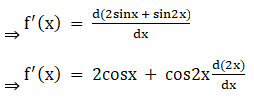⇒ f’(x) = 2cosx + 2cos2x

⇒ f’(x) = 2cosx + 2(2cos2x – 1)

⇒ f’(x) = 4 cos2x + 2 cos x – 2

We have f’(c) = 0,

⇒ 4cos2c + 2 cos c – 2 = 0

⇒ 2cos2c + cos c – 1 = 0

⇒ 2cos2c + 2 cos c – cos c – 1 = 0

⇒ 2 cos c (cos c + 1) – 1 (cos c + 1) = 0

⇒ (2cos c – 1) (cos c + 1) = 0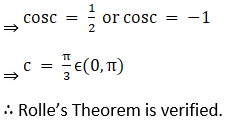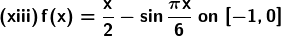Solution: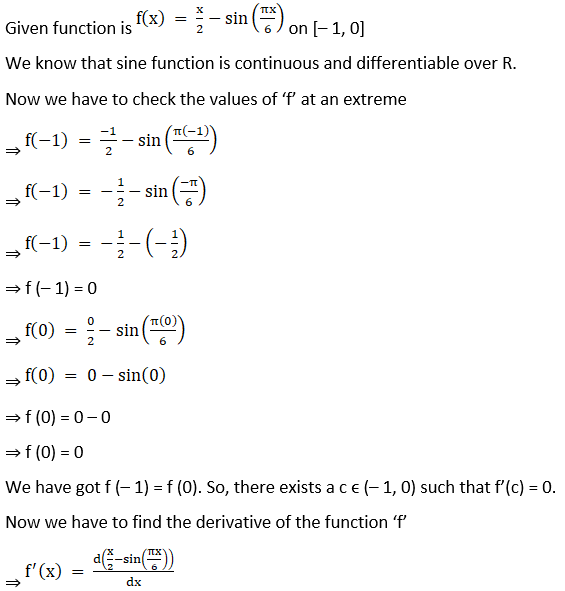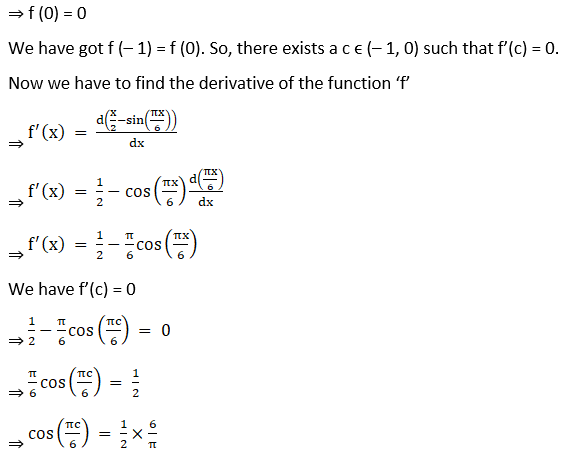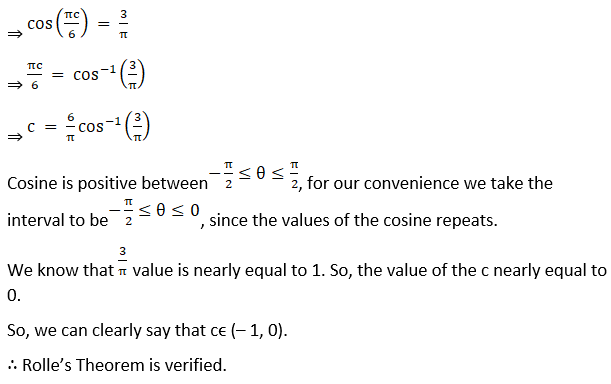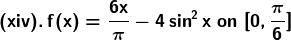Solution: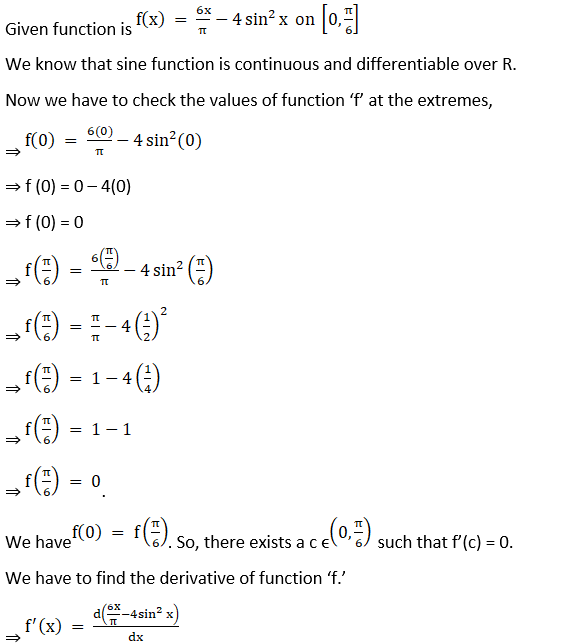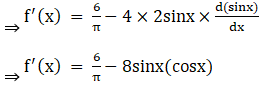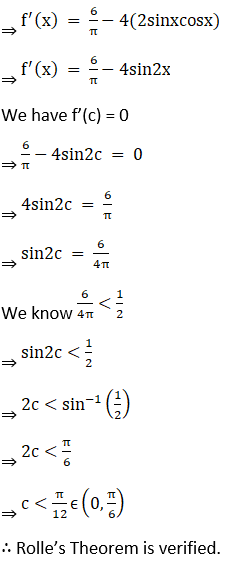(xv) f (x) = 4sin x on [0, π]

Solution: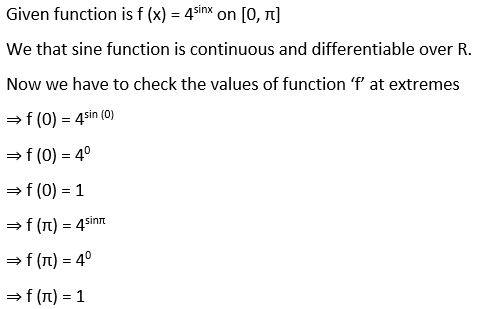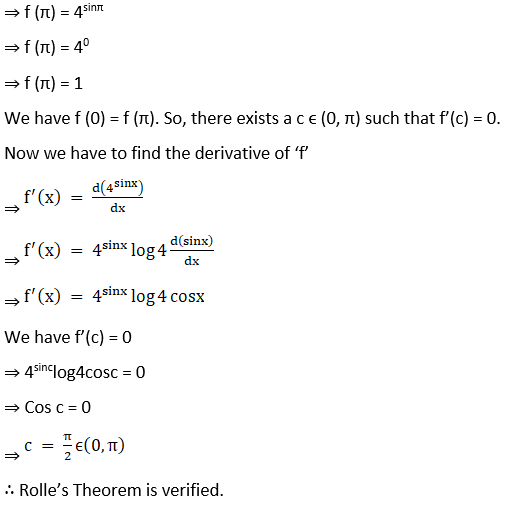(xvi) f (x) = x2 – 5x + 4 on [0, π/6]

Solution:

Given function is f (x) = x2 – 5x + 4 on [1, 4]

Since the given function f is a polynomial, it is continuous and differentiable everywhere, i.e., on R.

Let us find the values at extremes

⇒ f (1) = 12 – 5(1) + 4

⇒ f (1) = 1 – 5 + 4

⇒ f (1) = 0

⇒ f (4) = 42 – 5(4) + 4

⇒ f (4) = 16 – 20 + 4

⇒ f (4) = 0

We have f (1) = f (4). So, there exists a c ϵ (1, 4) such that f’(c) = 0.

Let’s find the derivative of f(x):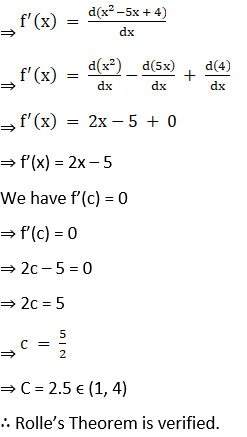(xvii) f (x) = sin4 x + cos4 x on [0, π/2]

Solution: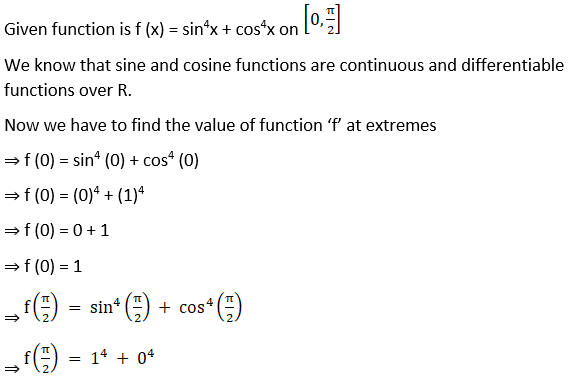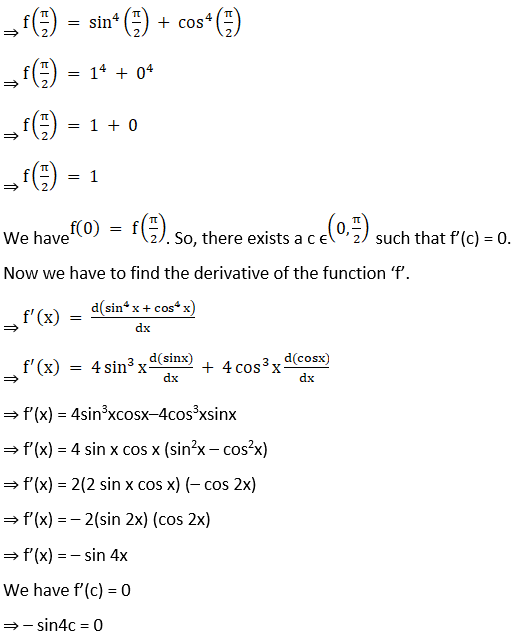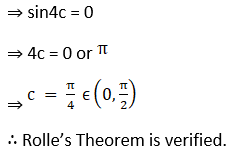(xviii) f (x) = sin x – sin 2x on [0, π]

Solution:

Given function is f (x) = sin x – sin2x on [0, π]

We know that the sine function is continuous and differentiable over R.

Now we have to check the values of the function ‘f’ at the extremes.

⇒ f (0) = sin (0)–sin 2(0)

⇒ f (0) = 0 – sin (0)

⇒ f (0) = 0

⇒ f (π) = sin(π) – sin2(π)

⇒ f (π) = 0 – sin(2π)

⇒ f (π) = 0

We have f (0) = f (π). So, there exists a c ϵ (0, π) such that f’(c) = 0.

Now we have to find the derivative of the function ‘f’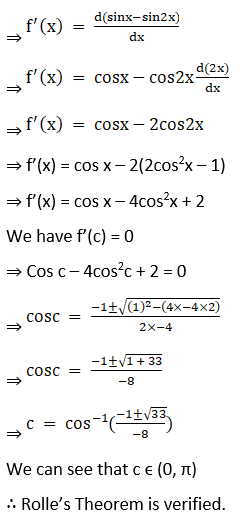4. Using Rolle’s Theorem, find points on the curve y = 16 – x2, x ∈ [-1, 1], where tangent is parallel to x – axis.

Solution:

Given function is y = 16 – x2, x ϵ [– 1, 1]

We know that the polynomial function is continuous and differentiable over R.

Let us check the values of ‘y’ at extremes

⇒ y (– 1) = 16 – (– 1)2

⇒ y (– 1) = 16 – 1

⇒ y (– 1) = 15

⇒ y (1) = 16 – (1)2

⇒ y (1) = 16 – 1

⇒ y (1) = 15

We have y (– 1) = y (1). So, there exists a c ϵ (– 1, 1) such that f’(c) = 0.

We know that for a curve g, the value of the slope of the tangent at a point r is given by g’(r).

Now we have to find the derivative of curve y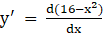⇒ y’ = – 2x

We have y’(c) = 0

⇒ – 2c = 0

⇒ c = 0 ϵ (– 1, 1)

The value of y at x = 1 is

⇒ y = 16 – 02

⇒ y = 16

∴ The point at which the curve y has a tangent parallel to the x-axis (since the slope of x – axis is 0) is (0, 16).